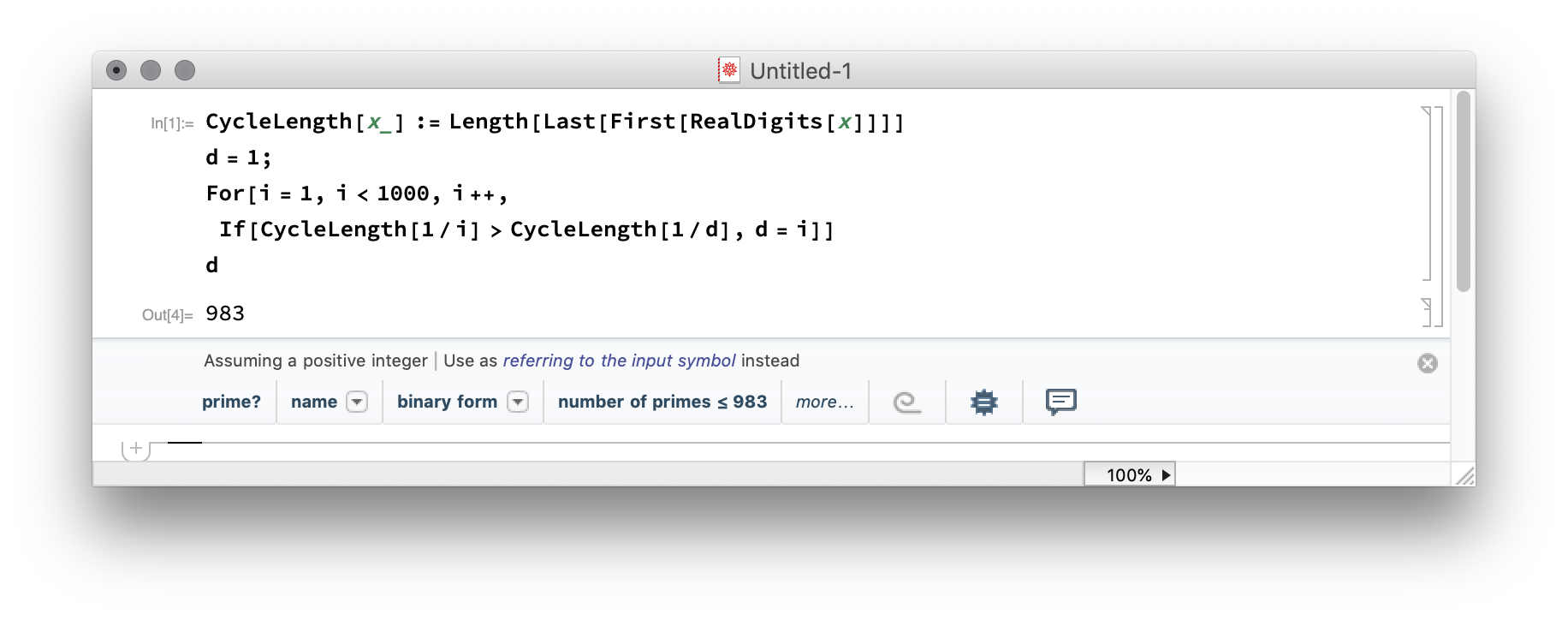# aliquote

## < a quantity that can be divided into another a whole number of time />

One of Euler problems deals with reciprocal cycles. When you write unit fractions using their decimal representation, you soon find that 1/3 which reads 0.33333 can be written as 0.(3) to indicate that it has a 1-digit recurring cycle. Likewise, 1/6 is simply 0.1(6), and so on. Eric Hanchrow provided a very elegant solution to solve this problem in Racket, by using coprime and a for/fold form. That’s what I ended up using (with little rewrite) because I was struggling with this problem for days until I came across this solution. I made up for that cheating by doing some research, especially digging a bit to better understand the role of coprimes in this specific case.

#lang racket

(require math/number-theory)

(define (lrcdf q)
(if (coprime? 10 q)
(unit-group-order 10 q)
0))

(for/fold ([p '(0 . 0)])
([x (in-range 2 1000)])
(let ([l (lrcdf x)])
(if (< (cdr p) l)
(cons x l)
p)))


As mentioned on the Racket docs, a set of integers is considered coprime if their greatest common divisor is 1. To put it in other words, any prime number that divides one does not divide the other.1 Since we are interested in decimal expansion with a viniculum, this means that we need to work with integers coprime to 10.

The key point resolves around full reptend prime. According to Wikipedia, a proper prime is a prime p which ends in the digit 1 in base 10 and whose reciprocal in base 10 has a repetend (i.e., repeating decimal segment) with length p − 1. Each digit appears in the repetend the same number of times, (p-1)/10. A prime is a proper prime if and only if it is a full reptend prime and congruent to 1 mod 10, i.e. $10^k \equiv 1\ (\text{mod}\ p)$ for $k=p-1, k\nless p-1$. As a side note, we could remark that if this prime number is also safe, that is a prime of the form 2p + 1 where p is also a prime, then 1/p will produce a stream of p − 1 pseudo-random digits. Anyway, the idea is to find the first full reptend prime number below a limit, here $d < 1000$.

I also happened to find a very nice solution in Mathematica, by Nayuki:2There’s more to see: The following fraction has 2997 recurring decimals!

$$\frac{1}{998001} = 0.000001002003004005…$$

Indeed, you will successively find all 3-decimal numbers from 000 to 999, until it starts over again at 000. Check out James Grime’s explanations on Numberphile.

1. Another interesting discussion on the use of modular arithmetic in testing the divisibility of a given number is available on Code Review. ↩︎

2. There’s also a GMP-based C version available on Github. ↩︎